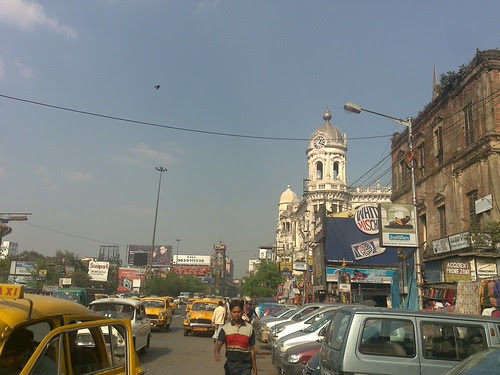## Monday, March 02, 2009

### Back To Basics: How does the GC find object referencesThis post is Part 9 in the series of posts on Garbage Collection (GC). Please see the index here.

Most garbage collection algorithms needs to know all other objects referenced by a given object to correctly traverse object hierarchy. All of reference counting, mark-sweep and even coping collectors need it. Consider the mark algorithm that I used in mark-sweep GC

`void Mark(Object* pObj){    if (!Marked(pObj)) // Marked returns the marked flag from object header    {        MarkBit(pObj); // Marks the flag in obj header        // Get list of references that the current object has        // and recursively mark them as well        ObjectCollection children = pObj->GetChildren();        for(int i = 0; i < children.Count(); ++i)        {            Mark(children[i]); // recursively call mark        }    }}`

Here a critical piece is the line in red. Given the object header we need to be able to locate all other objects referenced by it (or are children of it in object reference graph). There are two basic ways to do this

### Conservative GC

In case a GC is being plugged into an existing system in the form of a library and there is no easy way to modify the runtime then a conservative (as opposed to precise) system is used. A conservative GC considers every bit pattern on the heap to be a valid reference to another heap object. For the above case given a object header the GC needs to have the following basic information

1. The size of the object
2. Alignment of pointers (lets assume it to be 32bit for our case)

So once the GC gets a object pointer pObj it considers all 32bit patterns in between pObj and (pObj + sizeof(Object)) to be references to other child objects. It then uses some basic elimination like whether the value points inside the current heap for the system. If it doesn’t match any elimination logic then it is considered to be a reference.

Even though this approach seems a bit extreme, it works out well and the only flip side is that it returns false positives and hence some garbage which should’ve been collected is left out (e.g. because an int containing a value accidentally matches the address of an object on the heap).

### Precise GC

In precise GC the GC precisely knows every reference held in a given object. This is possible only if the runtime generates and preserves this information.

As a sample implementation I will describe how the .NET Compact Framework or .NETCF (which uses a precise garbage collector) goes doing about this.

One additional goal employed by .NETCF is that the GC should be independent of the type system (that is shouldn’t need to have any information of types of objects).

Every object in NETCF has an object header which in turn contains a pointer to it’s type descriptor or class descriptor. This class descriptor has a special 32-bit field named RefMap which is used to store reference information stored by a given type

So given an object pointer pObj the GC fetches the object header from before the pointer and then de-references the class descriptor pointer to get to the corresponding type’s Class descriptor. It then reads the RefMap stored into it. To do all of the above the GC needs to know only the object header and class descriptor layout and not any other type information.

All references in NETCF are 32-bit aligned and the refmap contains one bit per 32-bits of the object. If the bit is set it means that the corresponding 32-bit is a reference.

Let’s take the following class

`class MyType{    public char a;    public char b;    public AnotherType t1;    public int i;    public AnotherType t2;}`

The memory layout of the class would be something like

The first two chars take 16 bits each. After that there are 3, 32-bit entities of which t1 and t2 (1st and 3rd) are references to other objects but i is not.

So the GC needs to know that given this object the 32-bits from 32nd and 96th are actually references. This is exactly what is encoded in RefMap field. It contains the bit-pattern 00000000000000000000000000001010 where the LSB is for the first 32 bits of the object (which is a+b in this case) and so on.

Since bit 1 of refmap is set it means (pObj + 1 * 32) is a reference. If that value is non-NULL then the GC can just de-reference it to get to the t1 object reference held by pObj.

Obviously it means that the maximum size of object that RefMap can encode is 32*4 = 128bytes. No points for guessing how it handles objects larger than that :)